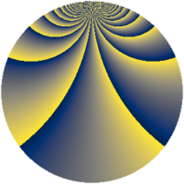# Properties

 Label 3549.2.efLevel $3549$ Weight $2$ Character orbit 3549.ef Rep. character $\chi_{3549}(44,\cdot)$ Character field $\Q(\zeta_{156})$ Dimension $23136$ Sturm bound $970$

# Related objects

## Defining parameters

 Level: $$N$$ $$=$$ $$3549 = 3 \cdot 7 \cdot 13^{2}$$ Weight: $$k$$ $$=$$ $$2$$ Character orbit: $$[\chi]$$ $$=$$ 3549.ef (of order $$156$$ and degree $$48$$) Character conductor: $$\operatorname{cond}(\chi)$$ $$=$$ $$3549$$ Character field: $$\Q(\zeta_{156})$$ Sturm bound: $$970$$

## Dimensions

The following table gives the dimensions of various subspaces of $$M_{2}(3549, [\chi])$$.

Total New Old
Modular forms 23520 23520 0
Cusp forms 23136 23136 0
Eisenstein series 384 384 0

## Trace form

 $$23136q - 22q^{3} - 52q^{4} - 88q^{6} - 102q^{7} - 22q^{9} + O(q^{10})$$ $$23136q - 22q^{3} - 52q^{4} - 88q^{6} - 102q^{7} - 22q^{9} - 52q^{10} - 26q^{12} - 192q^{13} - 92q^{15} - 996q^{16} - 46q^{19} - 46q^{21} - 384q^{22} - 46q^{24} - 52q^{25} - 88q^{27} - 68q^{28} - 130q^{30} - 38q^{31} - 10q^{33} - 256q^{34} - 104q^{36} - 46q^{37} - 98q^{39} + 60q^{40} - 56q^{42} - 208q^{43} - 24q^{45} + 132q^{46} - 80q^{48} - 104q^{49} - 26q^{51} - 36q^{52} - 40q^{54} - 176q^{55} - 60q^{57} - 24q^{58} - 70q^{60} - 44q^{61} - 214q^{63} - 208q^{64} - 120q^{66} - 258q^{67} + 52q^{69} + 148q^{70} - 14q^{73} + 104q^{75} - 200q^{76} - 144q^{78} - 92q^{79} - 46q^{81} - 52q^{82} + 106q^{84} - 288q^{85} - 2q^{87} - 260q^{88} - 104q^{90} - 130q^{91} - 30q^{93} - 240q^{94} + 100q^{96} - 208q^{97} - 100q^{99} + O(q^{100})$$

## Decomposition of $$S_{2}^{\mathrm{new}}(3549, [\chi])$$ into newform subspaces

The newforms in this space have not yet been added to the LMFDB.